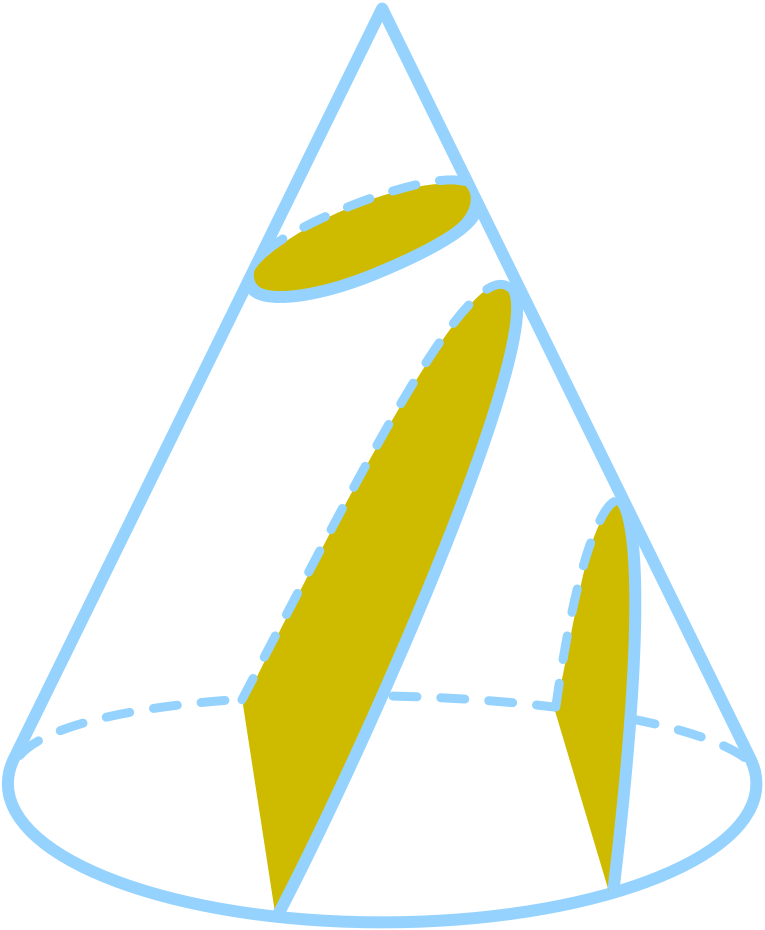# Circles to Polar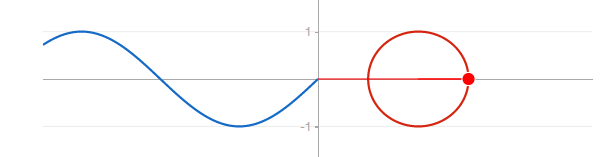The previous quiz started with the idea of similar right triangles, and led to the sine function which traces the path of a circle.

This quiz further develops the connection between circles and right triangles, leading to a much more elegant way of representing circles that unlocks an entirely new way of looking at graphs!

## Circles to Polar

### Introduction

# Circles to Polar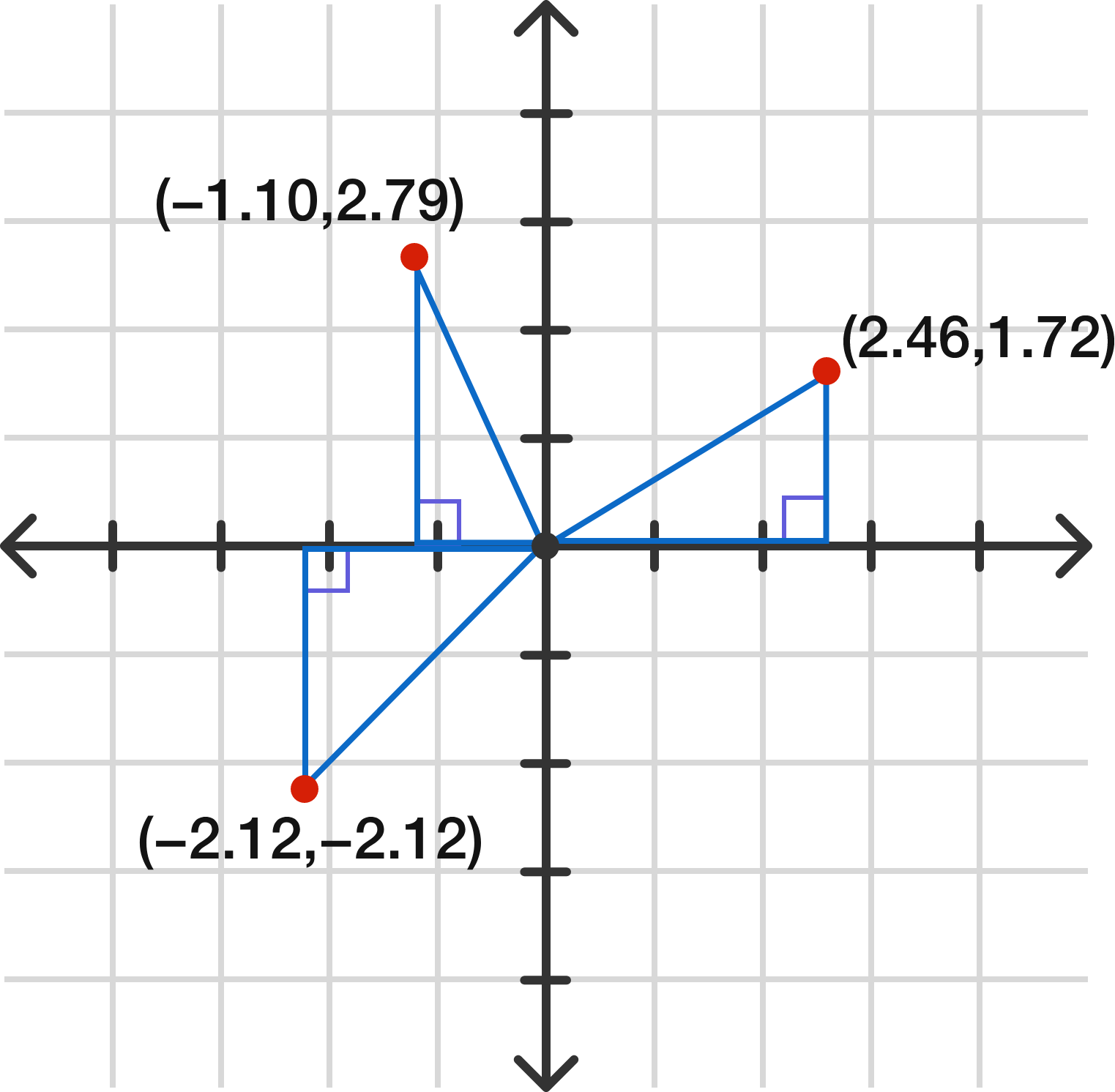The graph $$x^2 + y^2 = 9$$ can be considered the set of all solutions to the Pythagorean theorem $$a^2 + b^2 = c^2,$$ where $$c$$ is set at 3. Several points on the graph (with approximations) are shown above. For example, $$-1.10^2 + 2.79^2$$ is approximately 9.

What does the entire graph of $$x^2 + y^2 = 9$$ look like?

## Circles to Polar

### Introduction

# Circles to Polar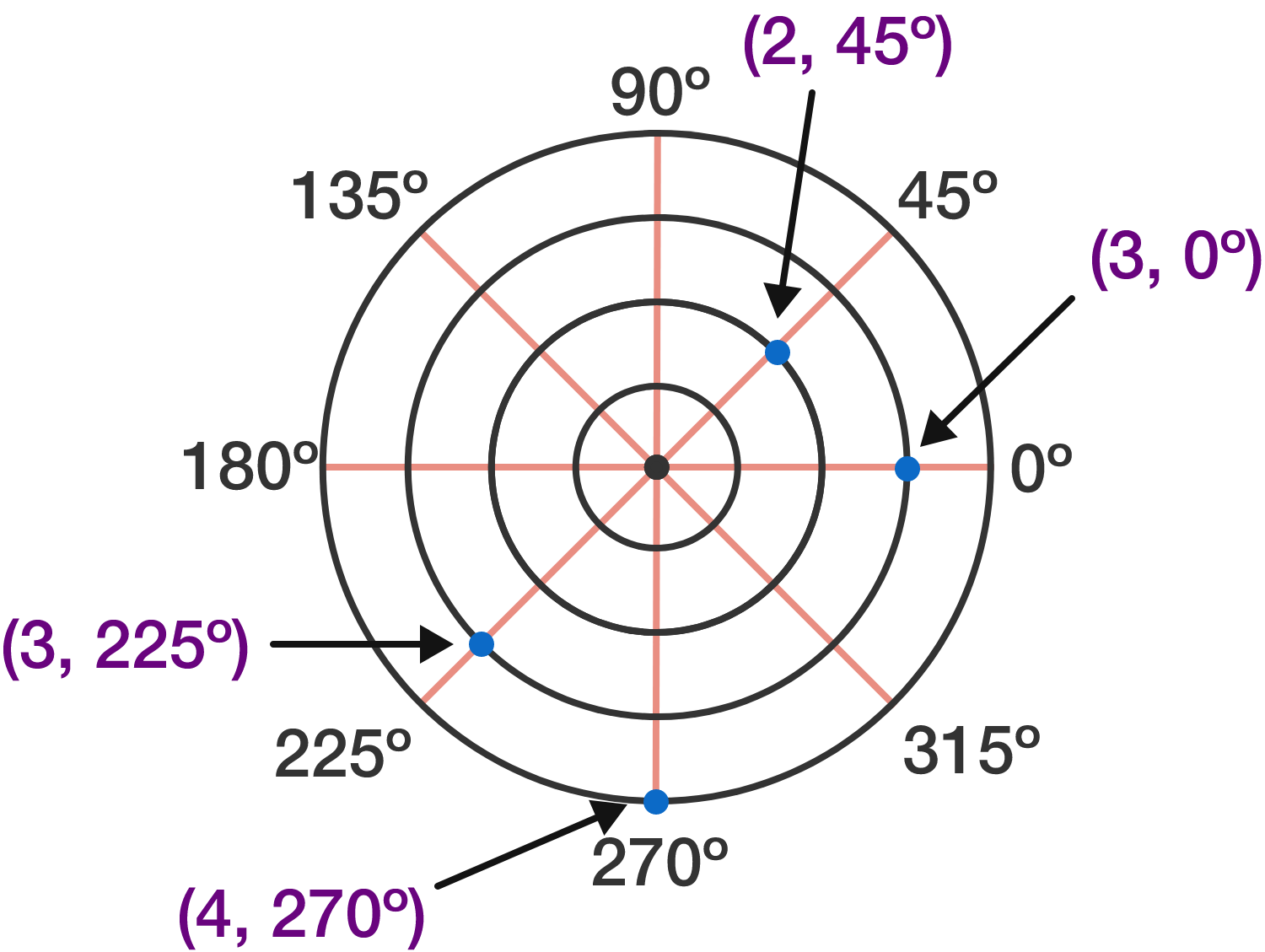In a traditional $$(x,y)$$-coordinate system from the previous problem, the first value $$x$$ indicates horizontal distance and the second value $$y$$ indicates vertical distance. In a polar coordinate system using $$(r, \theta),$$ the value $$r$$ gives "distance forward from origin when facing angle $$\theta.$$" Some example points are shown above.

One curious thing is that $$r$$ can be negative. Which point is $$(-3, 135^\circ) ?$$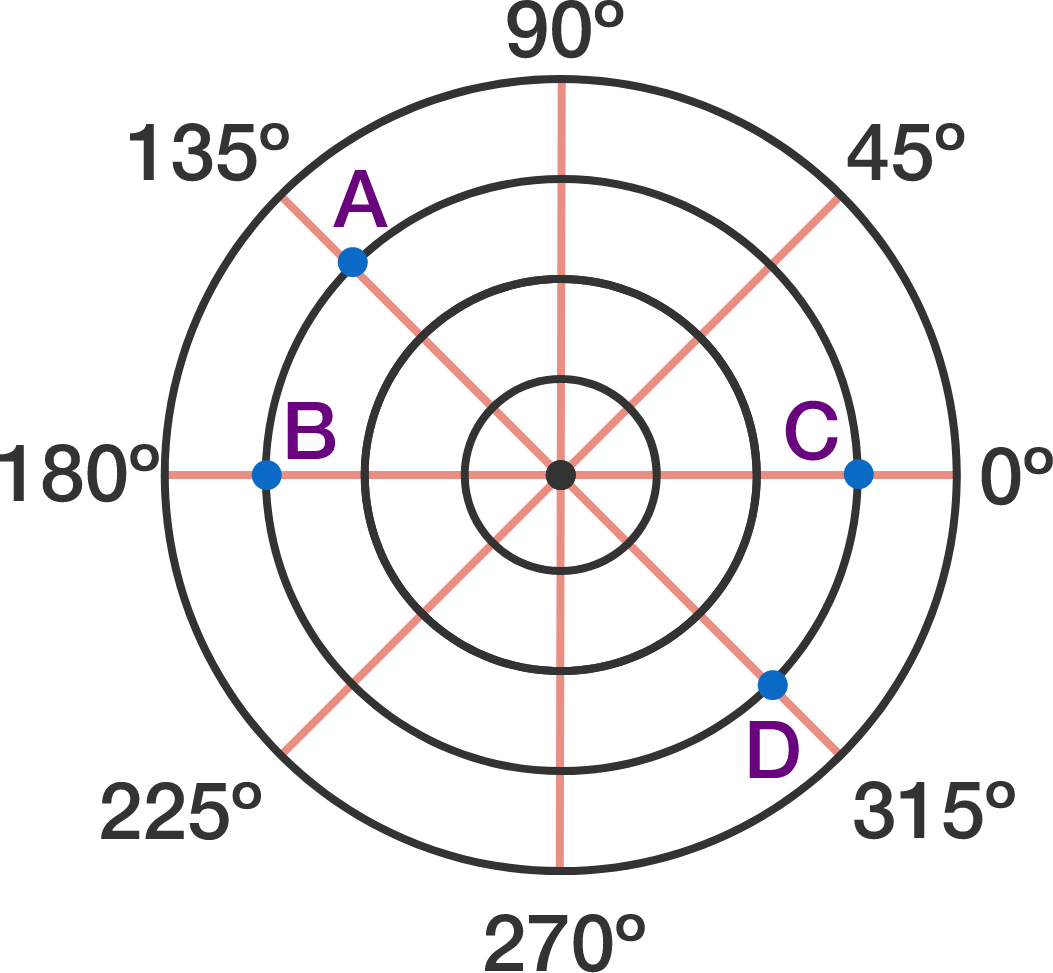## Circles to Polar

### Introduction

# Circles to Polar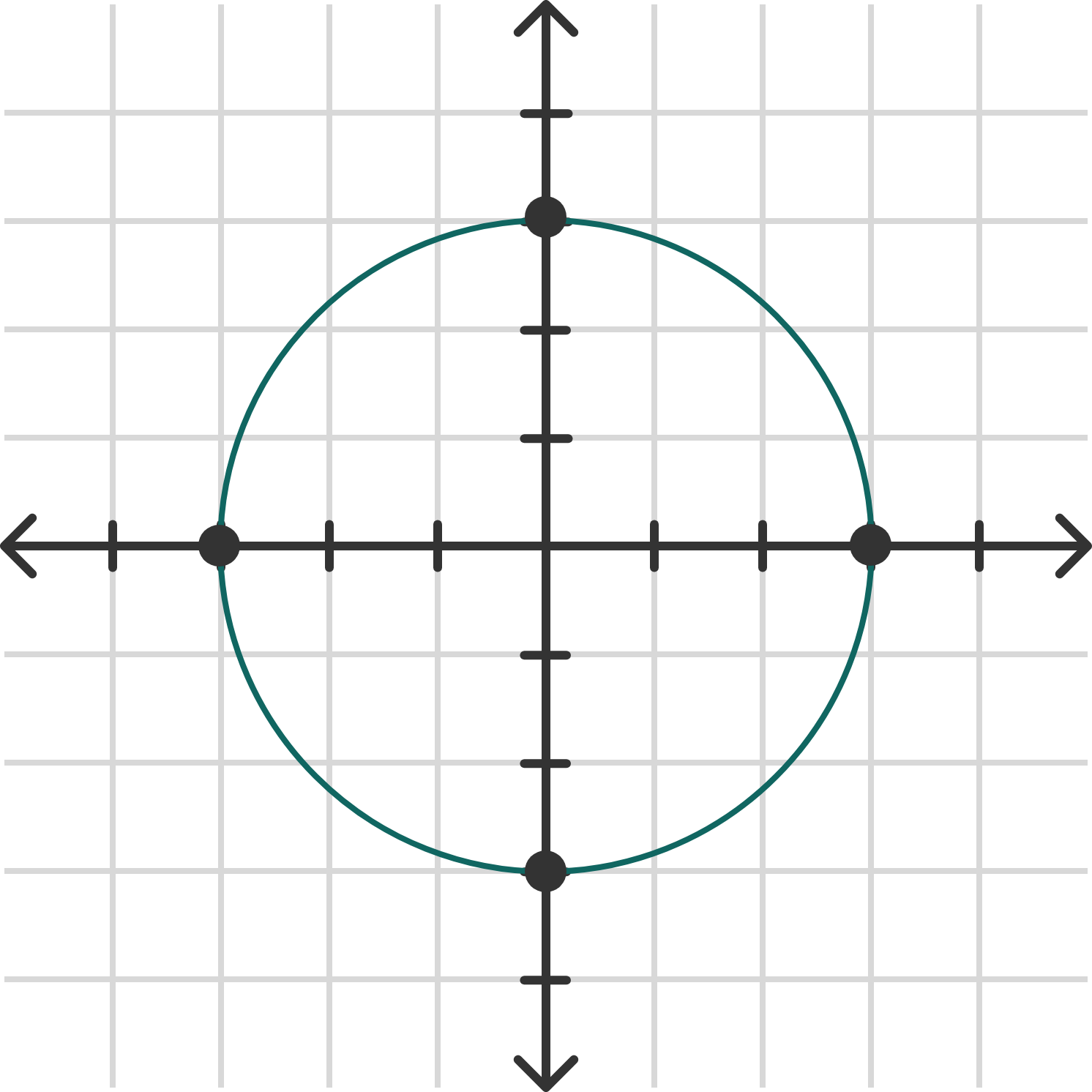We saw the graph of the circle above written as $$x^2 + y^2 = 9$$ in rectangular coordinates. How can you write it using polar coordinates? (The visualization below may help.)

## Circles to Polar

### Introduction

# Circles to Polar

Other than the same point being identified with a positive $$r$$ (facing forward) or a negative $$r$$ (facing backward), it's possible for the same point to be identified in an infinite number of ways via the measure of angle.

The point below can be identified as $$(2, 180^\circ) .$$ Which of the choices also identifies the same point?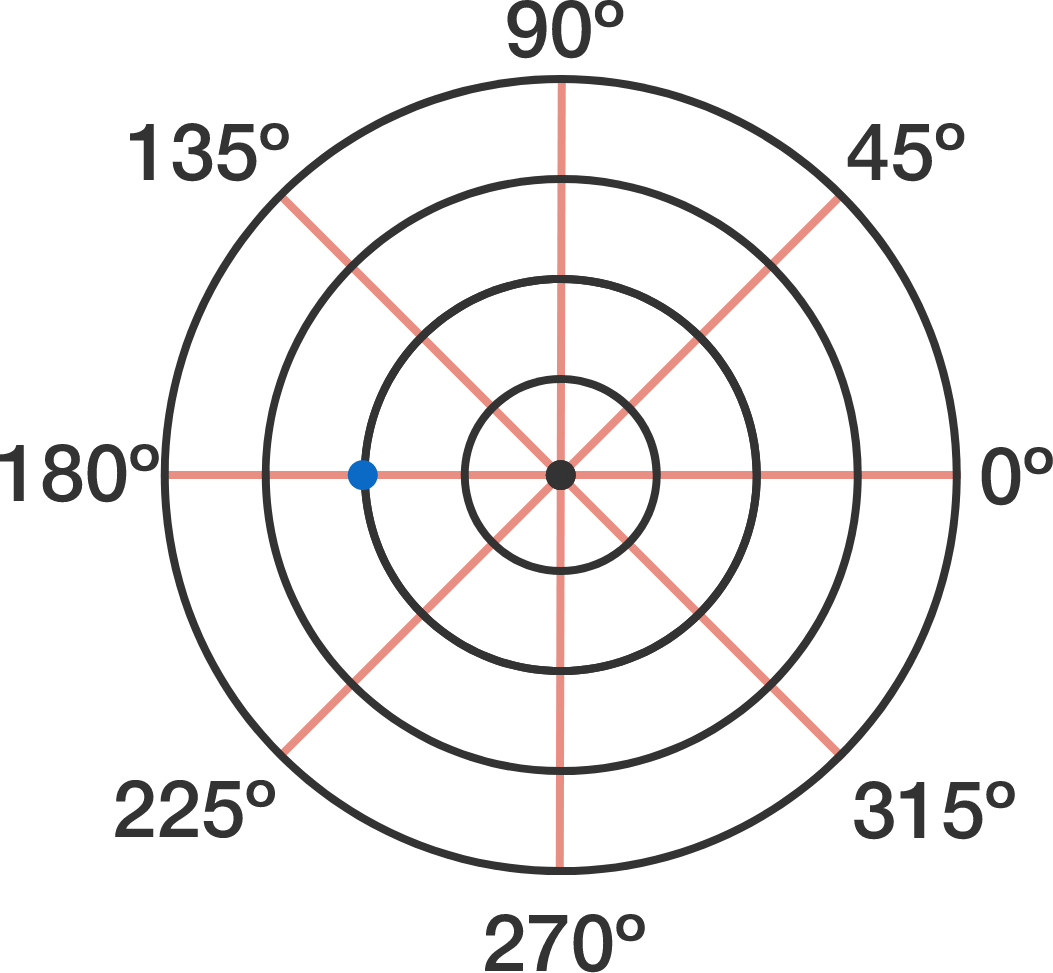## Circles to Polar

### Introduction

# Circles to Polar

Because of possible negative values, polar graphs are often restricted to particular values of $$r$$ and $$\theta .$$

If we restrict $$\theta$$ to positive values only, what kind of graph will $$r= \theta$$ be?

## Circles to Polar

### Introduction

# Circles to Polar

The visualization below lets you see the effect of $$b$$ in the spiral graph $$r = b \theta .$$

What will happen to the graph if $$b$$ is allowed to be negative?

## Circles to Polar

### Introduction

# Circles to Polar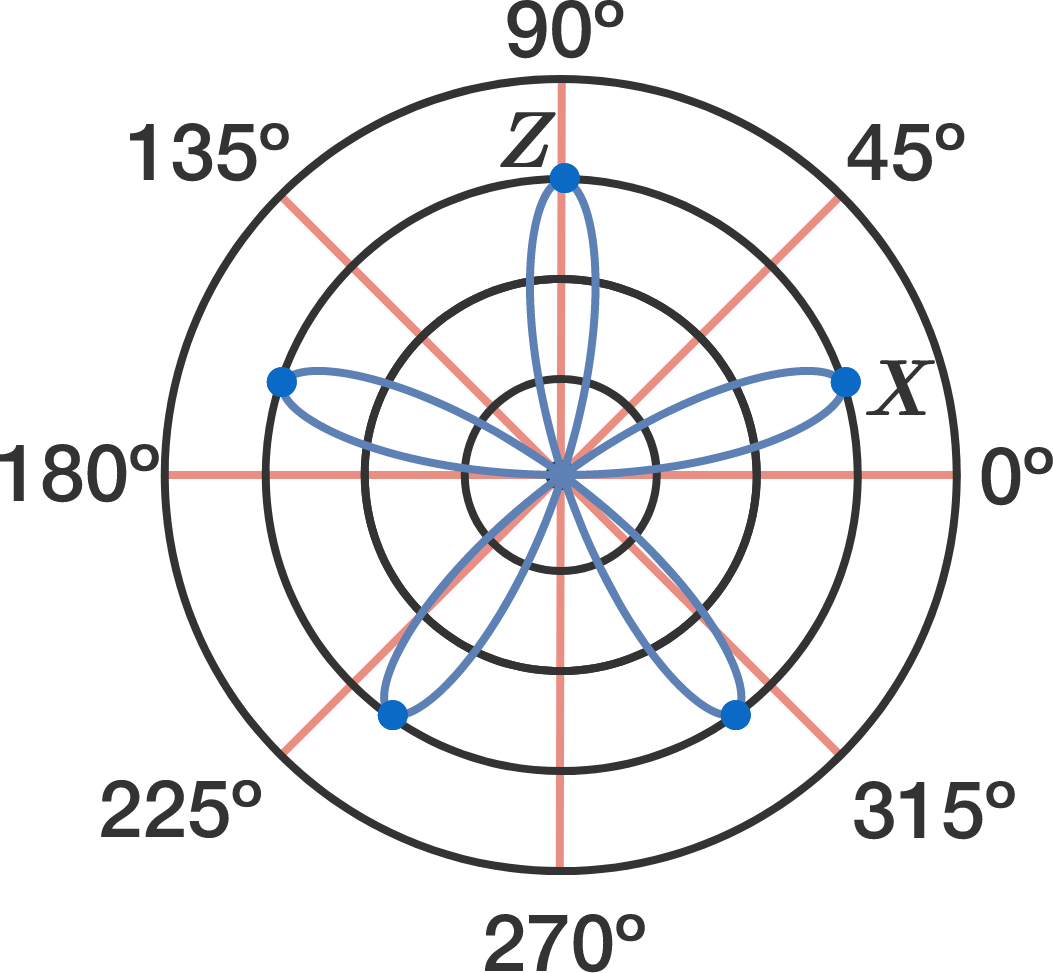As part of this course, you'll combine polar coordinates with trigonometry to get some spectacular graphs. The graph above involving the sine function is called a "rose" and has five equally spaced "petals."

If the point $$Z$$ is at $$(3, 90^\circ) ,$$ where is the point $$X?$$

## Circles to Polar

### Introduction

# Circles to Polar

The "rose" you just saw is represented by $$r = \sin(b\theta) ,$$ and you can experiment with it using the visualization above.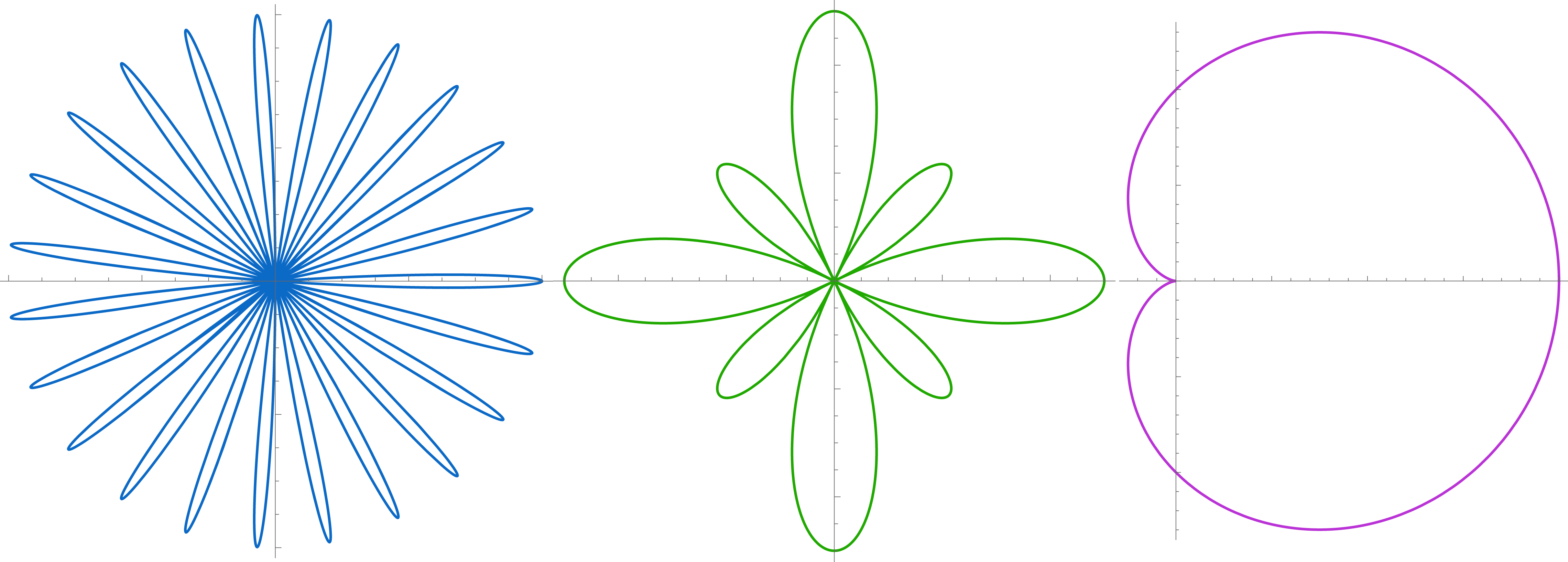Once you combine polar graphs with trigonometry, you can unlock very complex graphs which are very hard to represent with traditional rectangular function format. Some of the graphs even apply to science (the third one above, the "cardioid", is used with microphones), but for now we're returning back to circles to go in a different direction: into outer space.

## Circles to Polar

### Introduction

×